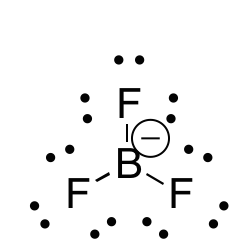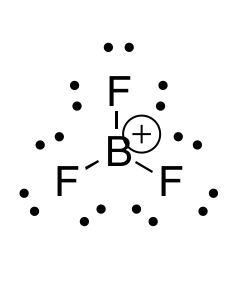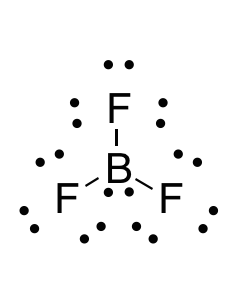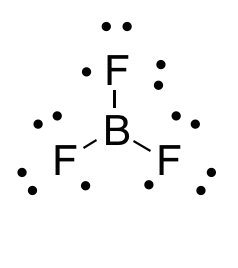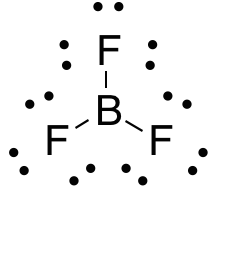# AP Chemistry : Lewis Diagrams

## Example Questions

### Example Question #41 : Compounds And Bonding

How many lone pairs of electrons exist on the following molecule?

BF3

10

9

1

11

0

9

Explanation:

..            ..

:F — B —  F:         Boron can have a violated octet (6 e-) and each F has 3 lone pairs

..      |      ..          for a total of 9 pairs of unpaired electrons

:F:

..

### Example Question #42 : Compounds And Molecules

Which of the following compounds contains an atom that does NOT satisfy the octet rule?

I.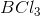II.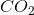III.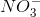IV.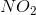II and III

IV only

I, II, and IV

I and IV

I and II

I and IV

Explanation:

BCl3 only has six electrons around boron, while NO2 (with an odd number of electrons) would have only 7 electrons around the central nitrogen.

### Example Question #43 : Compounds And Molecules

Which of the following elements will have two unpaired valence electrons?

Fluorine

Neon

Nitrogen

Carbon

Beryllium

Carbon

Explanation:

Lewis dot diagrams can help us keep track on how the valence electrons will disperse themselves among orbitals in the atom. When drawing these diagrams, it is important to keep two things in mind.

1. The s orbital will be filled before the p orbital begins to be filled.

2. Electrons will never pair in the same orbital if an orbital of equal energy is unoccupied.

Knowing these two facts, we can predict which of the following elements will have two unpaired electrons.

Fluorine will have seven valence electrons, meaning that only one orbital will not be completely filled.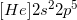Beryllium only has two valence electrons, but they will both be found in the 2s orbital, because it must be filled before the p orbitals can receive electrons.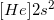Nitrogen will have five valence electrons, so two will be found in the 2s orbital. The other three will be present in their own p orbitals, meaning nitrogen has three unpaired electrons.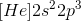Neon will have eight valence electrons, fully filling the 2s and 2p orbitals. It will have no unpaires electrons.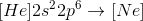Carbon has one less valence electron than nitrogen, meaning that it will have two unpaired electrons in the p orbitals.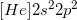### Example Question #41 : Compounds And Bonding

Which of the following is isoelectric with the cation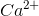?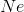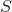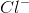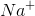Explanation:

Isoelectronic means having the same electron configuration (i.e. the same number of electrons).andboth have the same number of electrons (18), while sulfur has 16, phosphorous has 15, neon has 10, and the sodium cation has 10.

### Example Question #1 : Lewis Diagrams

Which answer option correctly depicts the Lewis dot structure of sodium chloride?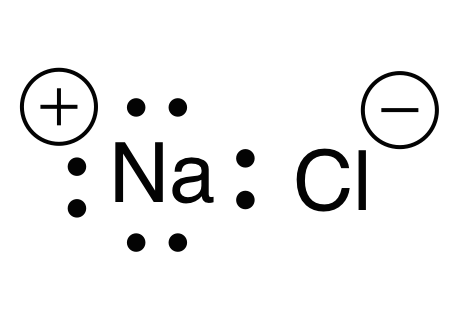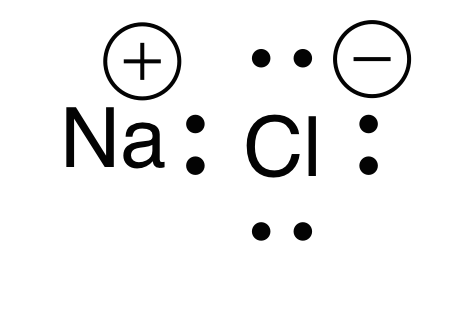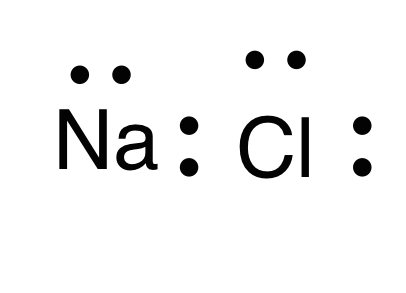Explanation:

When drawing a Lewis dot structure, we are always trying to reach an electron count where all atoms involved are stable and (usually) have full octets. We are also trying to estabilsh a structure in which we have the smallest formal charge possible. The general rule is first to draw out all of the elements involved and their valence electrons, then start piecing them together trying to reduce the formal charge and get all elements involved to an octet. There are a couple exceptions to the octet rule.

Sodium and chlorine form an ionic bond, meaning that one atom will donate an electron and the other will receive it. This gives each atom a charge. Chlorine has seven valence electrons, while sodium has one valence electron. For each atom to arrive at an octet, sodium will need to lose one electron and chlorine will need to gain one electron. This would give chlorine a negative charge, and sodium a positive charge.

Thus, the answer is a sodium with a positive charge (due to one lost electron) and a chlorine with eight electrons and a negative charge (due to one electron gained).

### Example Question #2 : Lewis Diagrams

What is the Lewis dot structure for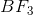?